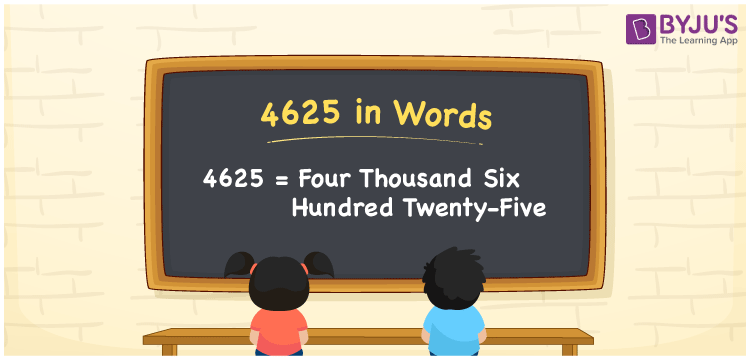# 4625 in Words

4625 in words can be written as Four Thousand Six Hundred Twenty-five. Students will be able to learn the conversion of 4625 in words which will help them understand the applications of numbers in our daily lives. If you buy a table for Rs. 4625, then you can say that “I have bought a table for Four Thousand Six Hundred Twenty-five Rupees”. The  number 4625 can be written in words using the English alphabet. The numbers in words can be grasped easily by the students using the resources given at BYJU’S. 4625 in English can be read as “Four Thousand Six Hundred Twenty-five”.

 4625 in words Four Thousand Six Hundred Twenty-five Four Thousand Six Hundred Twenty-five in Numbers 4625

## 4625 in English Words## How to Write 4625 in Words?

Students will learn about the conversion of 4625 into words from place value charts. The number 4625 has four digits. For 4625, the place value chart is prepared in a table form to help students understand it effectively.

 Thousands Hundreds Tens Ones 4 6 2 5

4625 in expanded form is explained in brief here:

4 × Thousand + 6 × Hundred + 2 × Ten + 5 × One

= 4 × 1000  + 6 × 100 + 2 × 10 + 5 × 1

= 4000 + 600 + 20 + 5

= 4625

= Four Thousand Six Hundred Twenty-five

Therefore, 4625 in words is written as Four Thousand Six Hundred Twenty-five.

4625 is a natural number that precedes 4626 and succeeds 4624.

4625 in words – Four Thousand Six Hundred Twenty-five

Is 4625 an odd number? – Yes

Is 4625 an even number? – No

Is 4625 a perfect square number? – No

Is 4625 a perfect cube number? – No

Is 4625 a prime number? – No

Is 4625 a composite number? – Yes

## Frequently Asked Questions on 4625 in Words

Q1

### How do you write 4625 in words?

4625 can be written as “Four Thousand Six Hundred Twenty-five” in words.
Q2

### Is 4625 an even number?

4625 is an odd number because it is not divisible by 2.
Q3

### How can Four Thousand Six Hundred Twenty-five be written in numbers?

Four Thousand Six Hundred Twenty-five can be written in numbers as 4625.